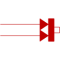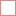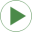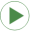# FixedHeatFlow

Fixed heat flow boundary condition# Information

This information is part of the Modelica Standard Library maintained by the Modelica Association.

This model allows a specified amount of heat flow rate to be "injected" into a thermal system at a given port. The constant amount of heat flow rate Q_flow is given as a parameter. The heat flows into the component to which the component FixedHeatFlow is connected, if parameter Q_flow is positive.

If parameter alpha is <> 0, the heat flow is multiplied by (1 + alpha*(port.T - T_ref)) in order to simulate temperature dependent losses (which are given with respect to reference temperature T_ref).

# Parameters (3)

Q_flow Value: Type: HeatFlowRate (W) Description: Fixed heat flow rate at port Value: 293.15 Type: Temperature (K) Description: Reference temperature Value: 0 Type: LinearTemperatureCoefficient (¹/K) Description: Temperature coefficient of heat flow rate

# Connectors (1)

 portType: HeatPort_b

# Used in Examples (3)HeatingSystem Modelica.Fluid.Examples Simple model of a heating systemIncompressibleFluidNetwork Modelica.Fluid.Examples Multi-way connections of pipes and incompressible medium modelBranchingDynamicPipes Modelica.Fluid.Examples Multi-way connections of pipes with dynamic momentum balance, pressure wave and flow reversal